### 3.3.2.3 Growth Function

As already mentioned, some works explicitly assume a particular interpolation of the generalized concentration between two grid points, for instance an exponential variation. No such assumption was necessary to calculate the fluxes in the presented discretization. Nonetheless, the resulting interpolation is needed in some cases and will be investigated in this section.

First, a variable upper boundary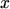is used in the definite integral (3.51)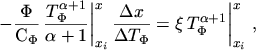(3.69)

which evaluates to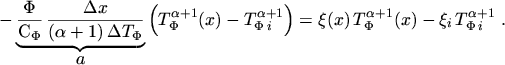(3.70)

By using eqn. (3.52) the coefficient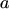is found to be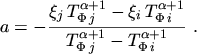(3.71)

A normalization of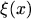to the range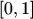can be achieved by using the substitution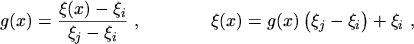(3.72)

where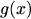is called growth function [8, p.156]. Inserting eqn. (3.71) and eqn. (3.72) into eqn. (3.70) yields the expression for the growth function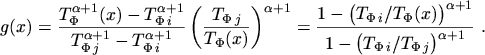(3.73)

A graph of this function with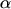as parameter is depicted in Fig. 3.5. The curves in the upper-left region are obtained for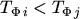and vice versa.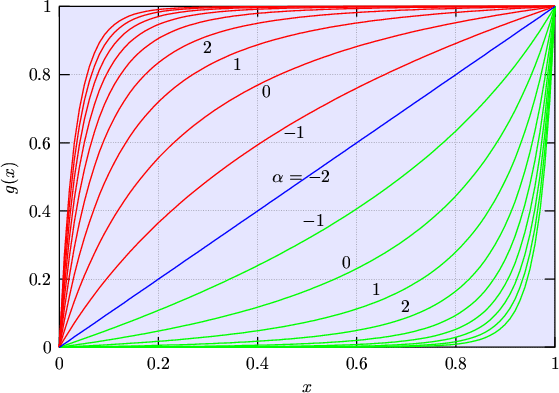M. Gritsch: Numerical Modeling of Silicon-on-Insulator MOSFETs PDF